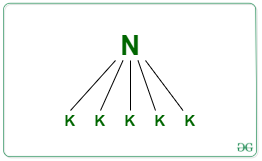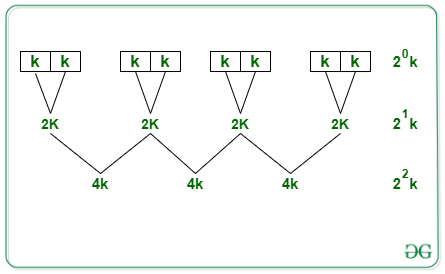Open in App
Not now

# Sorting by combining Insertion Sort and Merge Sort algorithms

• Difficulty Level : Hard
• Last Updated : 14 Feb, 2022

Insertion sort: The array is virtually split into a sorted and an unsorted part. Values from the unsorted part are picked and placed at the correct position in the sorted part.

• If the size of the list to be sorted is small, insertion sort runs faster
• Insertion sort takes O(N) time when elements are already sort
• It is an in-place algorithm O(1), no auxiliary space is required

Merge sort: Merge Sort is a Divide and Conquer algorithm. It divides the input array into two halves, calls itself for the two halves, and then merges the two sorted halves.

• The division of the main problem into sub-problem has no major cost

From the above two comparisons, the advantages of both the sorting algorithms can be combined, and the resulting algorithm will have time complexity O(N[K+log(N/K)]). Below is the derivation of the time complexity of this combined algorithm:
Let, no. of elements in the list = N
Divide:

• We first divide these N elements into (N/K) groups of size KSorting:

• For each division of subarray of size K, perform the insertion sort operation to sort this subarray
• The total cost of insertion sort for a single block of K elements:
• For best case: O(K)
• For worst case: O(• Since there are (N/K) such blocks each of size K, we get the total cost of applying insertion sort as:
• For best case: (N/K) * K = O(N)    <– (1)
• For worst case: (N/K) * K^{2} = O(NK)    <– (2)

Merging:

• After applying Insertion sort on (N/K) groups each of K sorted elements
• For merging these (N/K) groups:• Let’s say we take i iterations of merge sort. So, for the loop to stop we will need to equate as:
• (2^i) * K = N
• (2^i) = N/K
• i*log(2) = log(N/K)        Taking log on both sides
• i = log(N/K)
• Cost of merging = O(N)
• Total cost of merging = No. of iteration * Cost of iteration
• = log(N/K)*N
• = N*log(N/K)
• = O(N*Log(N/K))      <– (3)

The total cost of the algorithm (insertion + merge) is:

• Best case:  N+Nlog(N/K)             <– from (1) and (3)
• Worst case: NK + Nlog(N/K)       <– from (2) and (3)

If K = 1, then it is complete merge sort which is better in terms of time complexity
If K = N, then it is complete Insertion sort which is better in terms of space complexity

My Personal Notes arrow_drop_up